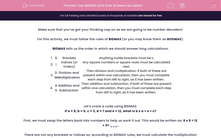# Use BIDMAS and Give Answers as Letters

In this worksheet, students will replace letters with numbers and solve problems using BIDMAS. They must give their final answers as letters not numbers.This content is premium and exclusive to EdPlace subscribers.Key stage:  KS 2

Curriculum topic:   Verbal Reasoning

Curriculum subtopic:   Maths Codes

Difficulty level:#### Worksheet Overview

Make sure that you’ve got your thinking cap on as we are going to be number decoders!

For this activity, we must follow the rules of BIDMAS (or you may know them as BODMAS).

BIDMAS tells us the order in which we should answer long calculations:

 1 B Brackets Anything inside brackets must be c 2 I Indices (or Orders) Any square numbers or square roots must be calculated next. 3 D  M Division and  Multiplication Then division and multiplication. If both of these are present within one calculation, then you must complete each step from left to right, as it has been written. 4 A  S Addition and  Subtraction Then addition and subtraction. If both of these are present within one calculation, then you must complete each step from left to right, as it has been written.

Let’s crack a code using BIDMAS:

If a = 8, b = 6, c = 3, d = 7 and e = 12, what is b x a ÷ e + c?

First, we must swap the letters back into numbers to help us work it out. This would be written as: 6 x 8 ÷ 12 + 3= ___.

There are not any brackets or indices so, according to BIDMAS rules, we must calculate the multiplication and division first. This must be done in the order that it is written, so 6 x 8 = 48.

Then we are left with: 48 ÷ 12 + 3.

Following BIDMAS, let’s do the division first, 48 ÷ 12 = 4.

Then we are left with 4 + 3 = 7.

So the answer is 7 but we must convert this back into a letter. The number 7 is represented by the letter d, therefore d is the final answer.

Let’s try one more and write the answer as a letter:

If a = 4, b = 125, c = 3, d = 11 and e = 7, what is d (c x a) - e?

Where there isn’t a -, +, x or ÷ between the first letter and the bracket, it means we have to multiply the outside number by what is in the brackets.

So the steps we follow are:

c x a = 12

d x 12 = 132

132 - e = 125

We must turn the number 125 back into a letter. This number corresponds to the letter b.

Now it’s your turn to crack the codes. Good luck number detective!

### What is EdPlace?

We're your National Curriculum aligned online education content provider helping each child succeed in English, maths and science from year 1 to GCSE. With an EdPlace account you’ll be able to track and measure progress, helping each child achieve their best. We build confidence and attainment by personalising each child’s learning at a level that suits them.

Get started